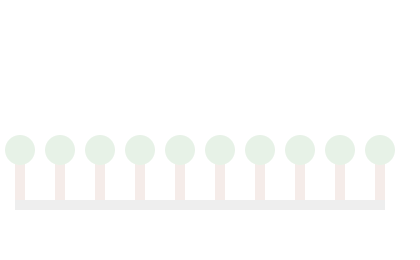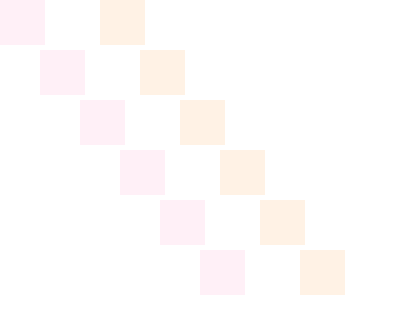## Overview

A while loop runs a block of code repeatedly until a condition evaluates to false.

Technically, while loops have two parts:

``````while (condition) {

// the code to do something

}``````

The `condition` is the expression evaluated at the start of each loop.

If the `condition` evaluates to true, the code block between the curly braces {…} is run and the loop starts again. If the `condition` evaluates to false, the while loop ends and the program continues.

Even though while loops technically only have two parts, some code inside the code block must eventually make the `condition` false, otherwise the loop will never end and your browser will hang or crash.

## Use a While Loop to Fit Text in a Box

In this example, we use a while loop to fit as many lines of text as possible in a box.

We start by declaring the variable `boxHeight`, which stores the height of the box, and initialize it with the value 200:

``var boxHeight = 200; // Assign the height of the box``

Then, we use the `context.fillRect()` method to draw a rectangle, using the value stored in the variable `boxHeight` for its height and filling it with the color `'Orchid'`. We also set the `context.fillStyle`, `context.font`, and `context.textBaseline` properties for our lines of text.

``````context.fillStyle = 'Orchid';
context.fillRect(0, 0, 300, boxHeight);

context.fillStyle = 'Black';
context.font = '16px Arial';
context.textBaseline = 'bottom';``````

We are using the variable `y` to store the y-coordinate of our lines of text. Before setting up the while loop, we declare the variable `y` and initialize it with the value 20, the y-coordinate of the first line of text.

``var y = 20; // Initialize the y-coordinate of the first line of text``

We want the while loop to continue running as long as the next line of text still fits inside of the rectangle. So, we use `y <= boxHeight` as our condition. If the y-coordinate of the next line of text is less than or equal to the height of the rectangle, we draw a line of text at the coordinates (10, `y`) and update the variable `y` with the y-coordinate for the next line of text.

``````while (y <= boxHeight) {
context.fillText('this line of text is at y = ' + y, 10, y);
y = y + 20; // Update the y-coordinate for the next line of text
}``````

Updating the value stored in the variable `y` inside of the while loop is important. If we don't do that, the while loop's condition will never evaluate to false and the loop will never end.

Change the value assigned to the variable `boxHeight` to see what happens. The while loop should draw just enough lines of text to fill the box. Count the lines of text to find the number of times the program ran through the loop.

Quick Reference:

Message Log

## Challenge 1

Update the while loop to draw trees from the start of the ground to the end, with trees 40 pixels apart. The ground starts at the x-coordinate stored in the variable `x1` and ends at the x-coordinate stored in the variable `x2`.

Quick Reference:

Message Log
##### Canvas (your drawing will display here)## Convert a For Loop into a While Loop

In this example, we convert a for loop into a while loop. To learn more about for loops, visit the For Loops lesson.

Most for loops and while loops have the same parts, just located in different places. In a for loop, we initialize, evaluate, and update the loop's condition all in the for statement.

``````for (var i = 0; i < 8; i = i + 1) {
context.fillRect(40 * i, 50, 36, 36);
}``````

This for loop draws eight squares in a row.

We can also set up a while loop to draw eight squares in a row. In a while loop, we still evaluate the loop's condition in the while statement, but we initialize the condition before the while statement and we update the condition inside the while loop's code block.

``````var j = 0; // Initialize the variable in the condition
while (j < 8) {
context.fillRect(40 * i, 150, 36, 36);
j = j + 1; // Update the condition by incrementing the variable
}``````

To help us compare the two loops, the squares in the for loop are filled with the color `'FireBrick'` and drawn at y = 50, and the squares in the while loop are filled with the color `'DarkSlateBlue'` and drawn at y = 150.

Quick Reference:

Message Log

## Challenge 2

The for loop below draws six squares in a diagonal line. Create a while loop to draw the same six squares, just shifted 100 pixels to right and filled with the color `'DarkOrange'` instead of `'HotPink'`.

Message Log
##### Canvas (your drawing will display here)## Fit Squares with Random Side Lengths along a Length

While loops and for loops both run a block of code repeatedly until a condition evaluates to false. We typically decide which kind of loop to use based on readability and clarity. Which kind of loop makes our code easier to follow and understand?

We generally use for loops if we know how many times the loop will repeat before starting to loop. If we know we are going to run through a loop six times, then initializing, evaluating, and updating the loop's condition in the for statement makes our code easier to read and follow. However, if we don't know how often the loop will repeat, using a while loop is often clearer.

In this example, we are going to fit as many squares as possible along a length. Because the size of the squares is random, there is no way to know ahead of time how many squares will fit.

We are using the variable `rowLength` to store the length for the row of squares and the variable `x` to store the x-coordinate of the next square. We start by declaring both variables and initialize `rowLength` with the value 360 and `x` with the value 0, the x-coordinate of the first square.

``````var rowLength = 360; // The length of the row of squares
var x = 0; // The x-coordinate of the next square``````

We want the while loop to continue running as long as the x-coordinate of the next square is still within the length of the row. So, we use `x < rowLength` as our condition. If the x-coordinate of the next square is less than the length of the row, we generate a random side length for the square, draw the square at the coordinates (`x`, 0), and update the variable `x` with the x-coordinate for the next square.

``````while (x < rowLength) {
var sideLength = 20 + 60 * Math.random(); // Generate a random side length between 20 and 80
context.fillRect(x, 0, sideLength, sideLength);
context.strokeRect(x, 0, sideLength, sideLength);
x = x + sideLength; // Calculate the x-coordinate of the next square
}``````

Run the program several times to see what happens.

There are a few things worth noting. First, we can count the number of squares to find the number of times the program ran through the loop. Second, the number of squares changes depending on the sizes of the squares. Third, the row of squares is longer than the `rowLength` because we are only checking the x-coordinate for the start of the square, not the end. Fourth, we calculate the x-coordinate of the next square using the value stored in the variable `sideLength`, which is a random number generated inside the while loop's code block. If we used `x = x + sideLength` to increment the variable `x` in a for statement, it wouldn't be clear where `sideLength` comes from. Doing the calculation inside the code block is clearer.

Quick Reference:

Message Log

## Challenge 3

Update the while loop to draw trees from the start of the ground to the end, with trees a random distance apart. The ground starts at the x-coordinate stored in the variable `x1` and ends at the x-coordinate stored in the variable `x2`. The random distance to the next tree is generated and stored in the variable `treeDistance` inside the while loop.

Run your program several times. Your drawings should look similar to the image to the right, except the number of trees and the distance between them should be different every time. Then, if your program seems to be working, mark the challenge as complete by selecting "Yes, it looks good".

Message Log

## Draw the Trajectory of a Cannonball

In this example, we use a while loop to draw the trajectory of a cannonball. We are using a while loop because the number of cannonballs drawn will vary depending on the angle of the cannon. Change the angle of the cannon to see the trajectory of the cannonball change.

We start by drawing the ground and using the angle of the cannon to calculate the geometry of the cannonball. We want to draw the first cannonball just as it is coming out of the barrel of the cannon. The angle of the cannon also determines the velocity of the cannonball in the x- and y-directions. Then, we draw the cannon itself at the coordinates (10, 300).

``````var angle = 30; // The angle of the cannon (10 - 80);

drawGround();
calculateGeometry();

context.translate(10, 300);
drawCannon();``````

By using the `context.translate()` method to position the cannon on the ground, we also move the origin of the context's coordinate system so the ground is at y = 0. This means, we can use `y < -5` as the condition for our while loop. As long as the cannonball is at least 5 pixels above the ground, we draw the cannonball and calculate the position and velocity of the next cannonball. The center of the cannonball needs to be at least 5 pixels above the ground because the radius of the cannonball is 5 pixels.

``````while (y < -5) { // Keep drawing the cannonball while it is above the ground
drawCannonball();

x = x + vX;
y = y + vY; // Calculate the coordinates of the next cannonball
vY = vY + g; // Calculate new velocity in the y-direction due to gravity
}``````

Inside the while loop, we use the velocity of the cannonball to calculate the coordinates of its next position, and then adjust the velocity of the cannonball due to the effect of gravity.

By using a while loop, we don't have to do any calculus or solve any quadratic equations in order to plot the trajectory of the cannonball ahead of time. We just keep drawing cannonballs as long as the position of the cannonball is still above the ground.